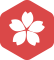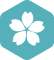### 怎么生成0-N的随机整数？

Web 开发 > JavaScript [问题点数：100分]2012年10月 Web 开发大版内专家分月排行榜第一
2012年9月 Web 开发大版内专家分月排行榜第一GitHub2012年10月 Web 开发大版内专家分月排行榜第一
2012年9月 Web 开发大版内专家分月排行榜第一JS随机生成0-20之间的随机整数，要求不重复

function abs() { var arr = new Array(); for(var i = 0; i < 10; i++) { //var n = Math.random() * 20 + 0; //n = Math.round(n);//四舍五入取整 var n = parseInt(...

Java基础-使用Math.random()生成指定范围随机整数

js生成指定范围的随机整数（例如0-100）

function random(min, max) { return Math.floor(Math.random() * (max - min)) + min; } for (var i = 1;... console.log(random(0, 100)); } 转载地址：https://blog.csdn.net/SundayAaron/art...

var ans = Math.floor(Math.random()*N)+1

python中生成随机整数_在Python中生成0到9之间的随机整数

python中生成随机整数Following are the few explanatory illustrations using different python modules, on how to generate random integers? Consider the scenario of generating the random numbers between 0...

python中随机生成数字_Python 中生成 0 到 9 之间的随机整数

java生成10以内的随机整数

import java.util.Random; public class RanTest { public static void main(String[] args) { ... for (int i=0;i<5;i++){ int random=new Random().nextInt(10); System.out.println(random); } } }

Java –生成范围内的随机整数

python3.6编写程序，生成20个0到100随机整数的列表，然后将前10个元素升序排列，后10个元素降序排列，并...

sql server 函数--rand() 生成随机整数

rand() 定义： 返回从0到1之间的随机浮点值。 举例说明： select rand()as随机数 结果如图： select cast(floor(rand()*N)as int) --方法1 结果：20 select cast( ceiling(rand()*N) as int ) --方法2 结果...

Python利用random模块随机生成浮点数、整数、字符串

我们处理一些问题时经常需要随机生成一些数据，这里介绍如何用python中的random模块生成浮点数、整数、字符串以及一些其他的小应用。 1.总结如下   (1) 随机的浮点数，范围是在0.0~1.0之间：random....

c++生成任意区间的随机整数随机整数序列

js获取1-100之间的随机整数

/* 获取随机整数 */ randomNum(m,n){ var num = Math.floor(Math.random()*(m - n) + n); return num; } /* 获取1-100之间的随机整数 */ var num=this.randomNum(1,101)； console.log( num ); ...

python练习--取随机整数

js求1到N之间的随机整数

randomIdenx = Math.floor(Math.random() * N);

java Math.random()生成n到m的随机整数

Java中Math类的random()方法可以生成...如果要生成[0,n]的随机整数的话，只需要Math.random()乘以n+1，生成[0,n+1)的浮点数，再强制类型转换为int类型，只取其整数部分，即可得到[0,n]的整数。int b=(int)(Math.ran...

python生成随机整数

python生成随机不重复的整数，用random中的sample index = random.sample(range(0,10),10) 上面是生成不重复的10个从1~10的整数 python生成完全随机整数，用numpy中的random.randint index = np....

js 生成两个整数之间不重复的n随机整数

Javascript或jQuery方法产生成指定范围90-100随机整数

python随机整数生成

import random #导入random包 print(random.randint(0,9)) #随机生成0-9之间的整数

MATLAB中如何生成指定范围的随机整数向量

1 致谢 感谢网友科比WILL的帮助， 原文链接如下： ...在做作业的时候遇到一个问题，需要生成一个不大于N随机整数向量，刚开始想到了rand()函数，不过感觉可能应该有更为简单的方法，所以咨询了一下度娘...

import java.util.Random; public class Test05 { public static void main(String[] args) { //Math方法 x即为[20,30]的整数。 int x = (int)(20+Math.random()*11); //Random方法 y即为[20-3...

javascript 生成0n随机数

randFrom0ToN=Math.floor(Math.random()*Math.floor(n)); 以上语句即可生成 0n 的随机数（整数